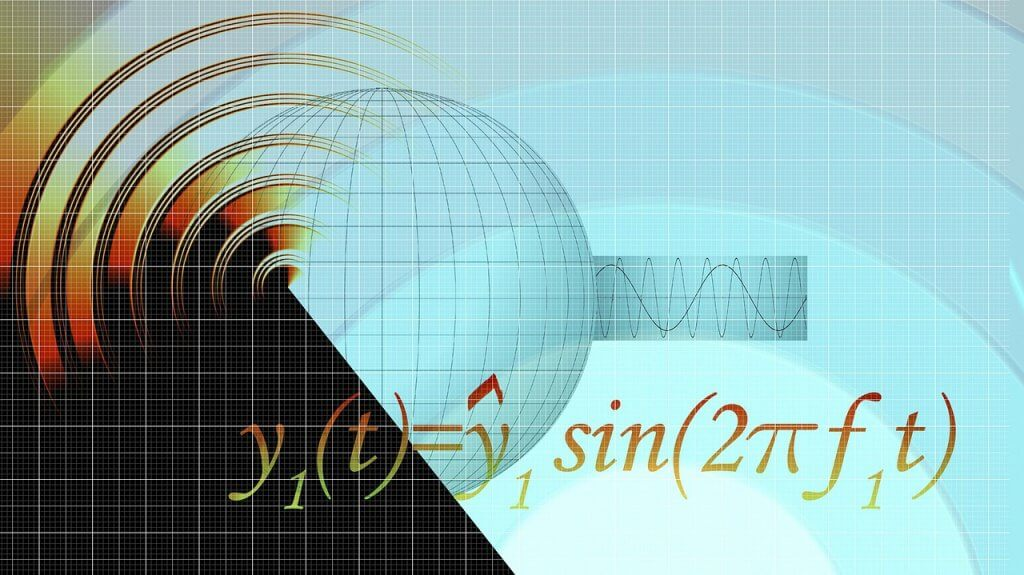# What is math

The notion that most people have of math is highly exaggerated. A lot of student’s passive math as a complicated subject that requires one to possess a genius mind for them to understand it. That is why when most students come across it, they are desperate for assistance. For homework solvers, do my math homework for me is a request they get very often.

## Meaning of math

So what is math? If you had asked me that question a while back, my answer would have been something like” math is about numbers, letters, and equations.” But after coming across the subject on various stages of my life, I learned that the definition of math is a science that deals with the logic of shape, quantity, and arrangement. Sounds complicated, right? Far from that, it is fascinating.

## Branches of math

I’m pretty sure you’re familiar with polynomial equations, trigonometry, calculus, differential equations, vectors, linear algebra, and many more. Some of these topics were introduced to you in middle school, high school through college. These are, among others, the subdivisions of mathematics. Mathematics is a broad subject that covers various areas of studies—grouped into arithmetic’s, geometry, algebra, analysis, and applied mathematics.
When we talk about geometry, it is the study of space. Therefore, when students learn topics like trigonometry, they are investigating the relations, properties, and measurements of surfaces, solids, angles, and lines. Arithmetic deals with the theory of numerical calculation; algebra focuses on the study of objects, and analysis focuses on the study of spaces with a topic such as calculus tackled.
Math provides solutions to problems in other areas such as computing, engineering, physics etc. This branch of math is called applied mathematics. It allows us to understand how mathematics applies to other fields of study. Hence there is more to math than just numbers. It is the building block of everything in our daily life. Math is a form of finding solutions to problems, justifying arguments, and a way of thinking. From mobile phones, money, architecture, engineering, and even sports, you name it, and math is involved.

### Conclusion

Its high time you start embracing math because it’s here to stay. Whether you like it or not, the fact is math is necessary for your day to day life. It may be complicated, but all you need is an interest in the subject and a little help with homework, and it will be among your favorite topics.